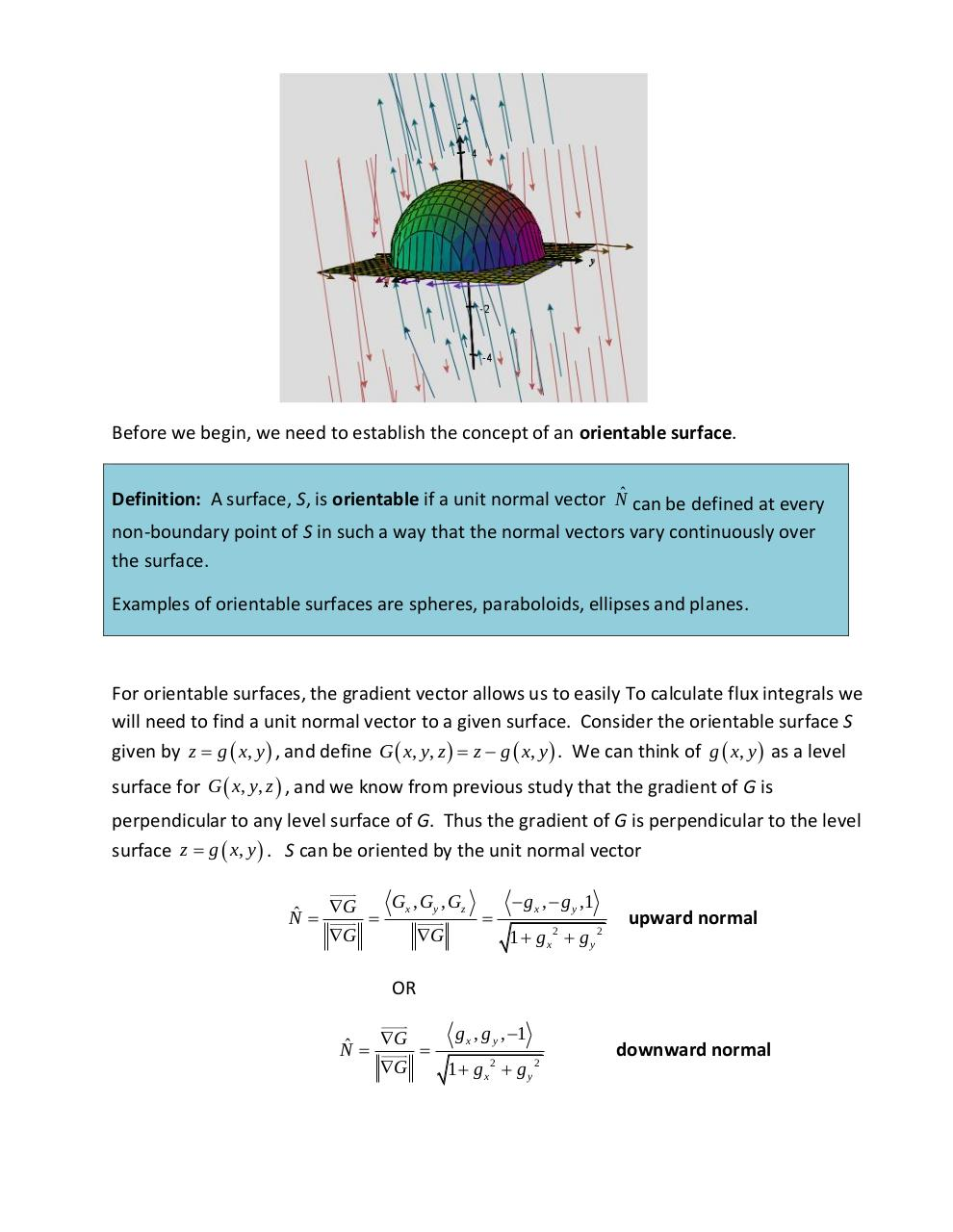# Lesson 10 6 Surface Integrals.pdfPage 1 2 3 4 5 6 7

#### Text preview

Before we begin, we need to establish the concept of an orientable surface.
Definition: A surface, S, is orientable if a unit normal vector Nˆ can be defined at every
non-boundary point of S in such a way that the normal vectors vary continuously over
the surface.
Examples of orientable surfaces are spheres, paraboloids, ellipses and planes.

For orientable surfaces, the gradient vector allows us to easily To calculate flux integrals we
will need to find a unit normal vector to a given surface. Consider the orientable surface S
given by z  g  x, y  , and define G  x, y, z   z  g  x, y  . We can think of g  x, y  as a level
surface for G  x, y, z  , and we know from previous study that the gradient of G is
perpendicular to any level surface of G. Thus the gradient of G is perpendicular to the level
surface z  g  x, y  . S can be oriented by the unit normal vector
Gx , Gy , Gz
 g x ,  g y ,1
G
Nˆ 

G
G
1  gx2  g y2

upward normal

OR
g x , g y , 1
G
Nˆ 

G
1  gx2  g y2

downward normal